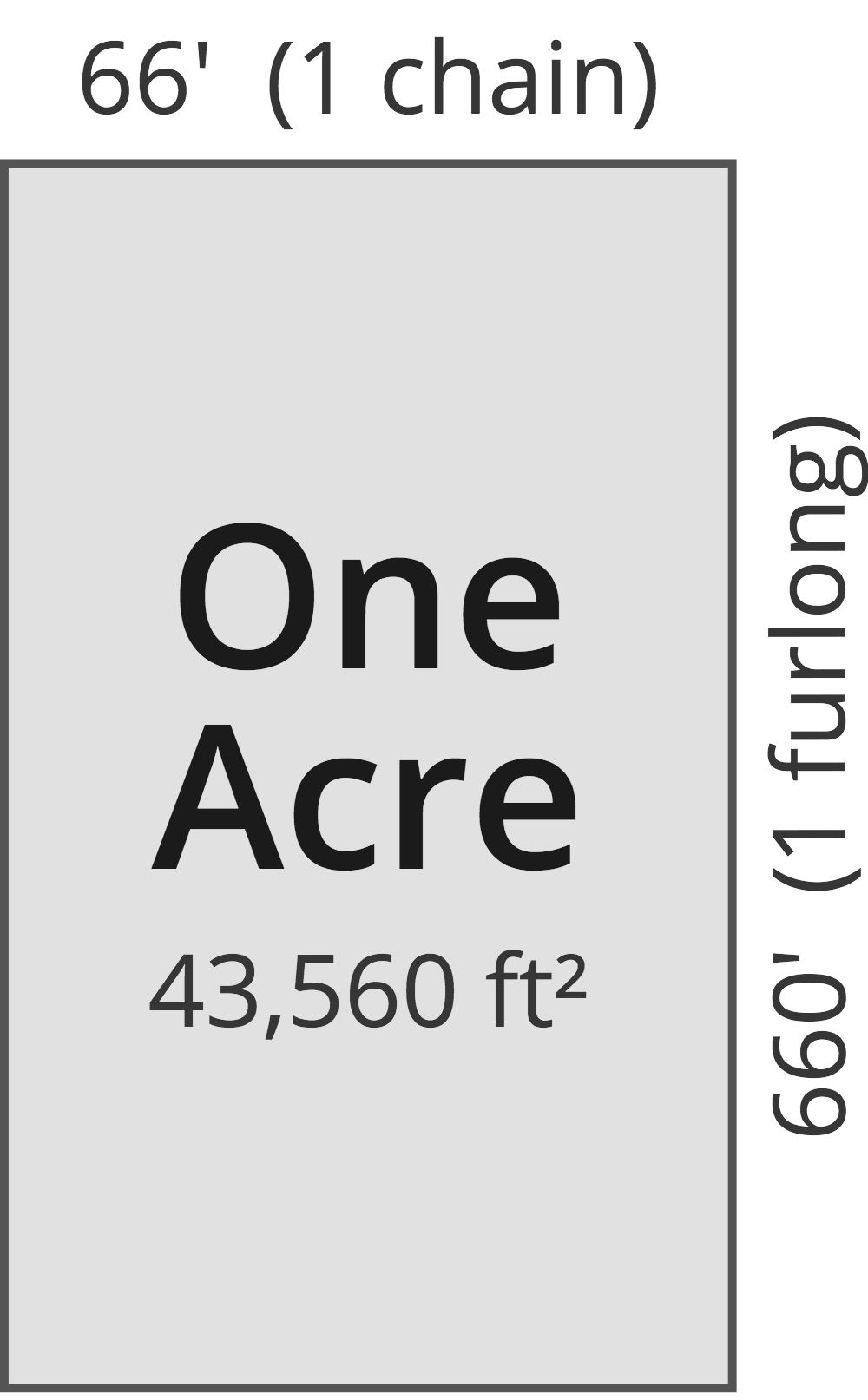# Acres to Square Feet Converter

Enter the area in acres below to get the value converted to square feet.

(find acres)
Results in Square Feet:1 ac = 43,560 sq ft

Do you want to convert square feet to acres?

## How to Convert Acres to Square Feet

To convert a measurement in acres to a measurement in square feet, multiply the area by the following conversion ratio: 43,560 square feet/acre.

Since one acre is equal to 43,560 square feet, you can use this simple formula to convert:

square feet = acres × 43,560

The area in square feet is equal to the area in acres multiplied by 43,560.

For example, here's how to convert 5 acres to square feet using the formula above.
square feet = (5 ac × 43,560) = 217,800 sq ft

### How Many Square Feet Are in an Acre?

There are 43,560 square feet in an acre, which is why we use this value in the formula above.

1 ac = 43,560 sq ft

## What Is an Acre?

One acre is defined as the area equal to a space that is one chain (66 ft) by one furlong (660 ft), or 10 square chains. That's equal to 43,560 square feet or 1/640 of a square mile for those unfamiliar with those units of measure.The acre is a US customary and imperial unit of area. Acres can be abbreviated as ac; for example, 1 acre can be written as 1 ac.

You can use an acreage calculator to measure the area of a plot of land in acres by locating the boundaries on a map.

## What Is a Square Foot?

One square foot is equivalent to the area of a square with sides that are each 1 foot in length. One square foot is equal to 144 square inches or 0.092903 square meters .

The square foot is a US customary and imperial unit of area. A square foot is sometimes also referred to as a square ft. Square feet can be abbreviated as sq ft, and are also sometimes abbreviated as ft². For example, 1 square foot can be written as 1 sq ft or 1 ft².

You can use a square footage calculator to calculate the area of a space if you know its dimensions.

## Acre to Square Foot Conversion Table

Table showing various acre measurements converted to square feet.
Acres Square Feet
0.0001 ac 4.356 sq ft
0.0002 ac 8.712 sq ft
0.0003 ac 13.07 sq ft
0.0004 ac 17.42 sq ft
0.0005 ac 21.78 sq ft
0.0006 ac 26.14 sq ft
0.0007 ac 30.49 sq ft
0.0008 ac 34.85 sq ft
0.0009 ac 39.2 sq ft
0.001 ac 43.56 sq ft
0.002 ac 87.12 sq ft
0.003 ac 130.68 sq ft
0.004 ac 174.24 sq ft
0.005 ac 217.8 sq ft
0.006 ac 261.36 sq ft
0.007 ac 304.92 sq ft
0.008 ac 348.48 sq ft
0.009 ac 392.04 sq ft
0.01 ac 435.6 sq ft
0.02 ac 871.2 sq ft
0.03 ac 1,307 sq ft
0.04 ac 1,742 sq ft
0.05 ac 2,178 sq ft
0.06 ac 2,614 sq ft
0.07 ac 3,049 sq ft
0.08 ac 3,485 sq ft
0.09 ac 3,920 sq ft
0.1 ac 4,356 sq ft
0.2 ac 8,712 sq ft
0.3 ac 13,068 sq ft
0.4 ac 17,424 sq ft
0.5 ac 21,780 sq ft
0.6 ac 26,136 sq ft
0.7 ac 30,492 sq ft
0.8 ac 34,848 sq ft
0.9 ac 39,204 sq ft
1 ac 43,560 sq ft

## References

1. National Institute of Standards and Technology, Specifications, Tolerances, and Other Technical Requirements for Weighing and Measuring Devices, Handbook 44 - 2019 Edition, https://nvlpubs.nist.gov/nistpubs/hb/2019/NIST.HB.44-2019.pdf
2. Merriam-Webster, square foot, https://www.merriam-webster.com/dictionary/square%20foot